Reading- Multiplication Using the Standard Algorithm | 3rd, 4th & 5th Grade
1%
It was processed successfully!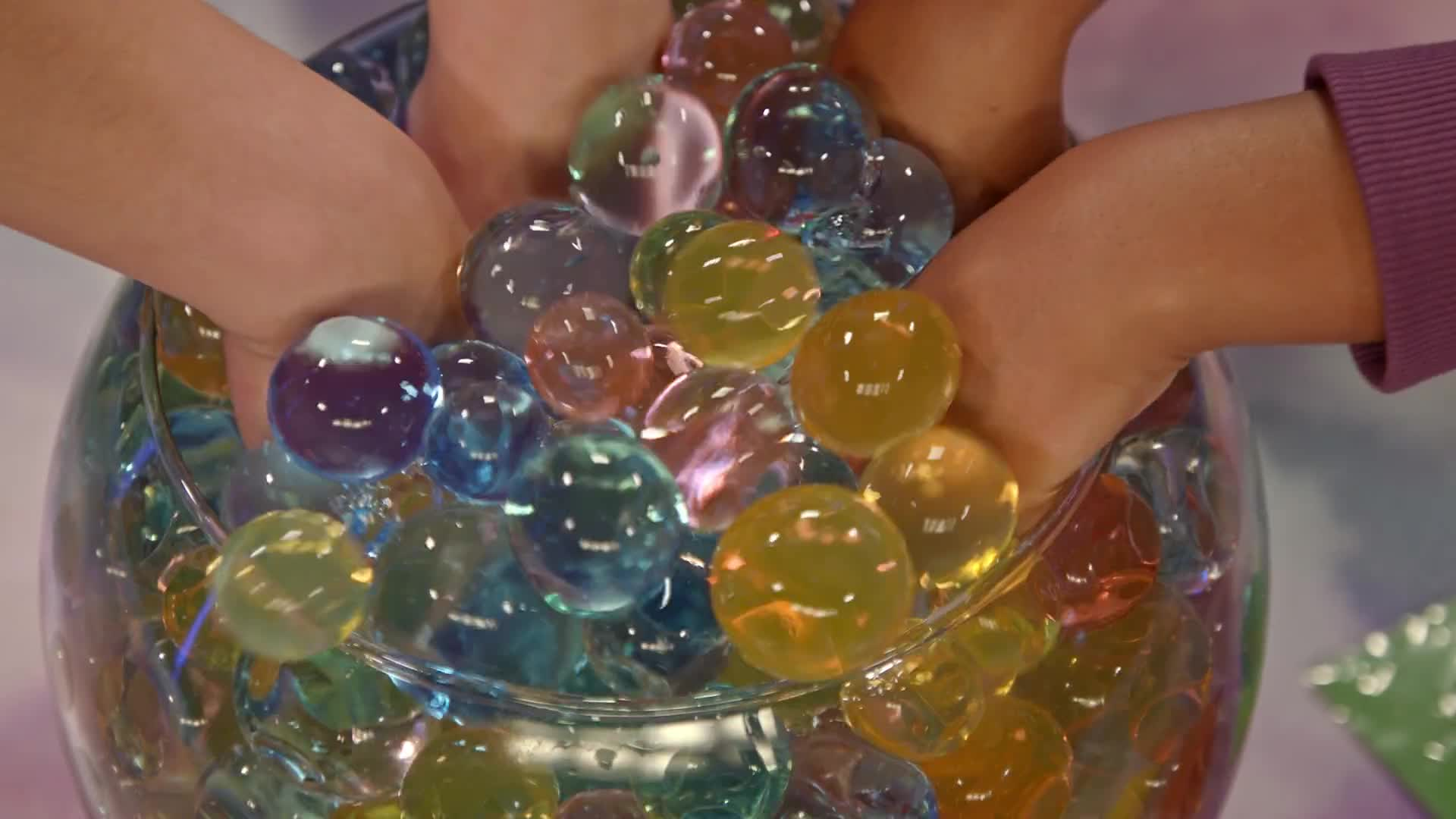WHAT IS MULTIPLICATION USING THE STANDARD ALGORITHM?

Warm up by recalling how to multiply using the area model method. You will then learn the multiplication algorithm, first with 3 digits × 1 digit, 4 digits × 1 digit, then 2 × 2 and 3 × 2.

To better understand multiplication using the standard algorithm…

WHAT IS MULTIPLICATION USING THE STANDARD ALGORITHM?. Warm up by recalling how to multiply using the area model method. You will then learn the multiplication algorithm, first with 3 digits × 1 digit, 4 digits × 1 digit, then 2 × 2 and 3 × 2. To better understand multiplication using the standard algorithm…

## LET’S BREAK IT DOWN!

### Jelly Balls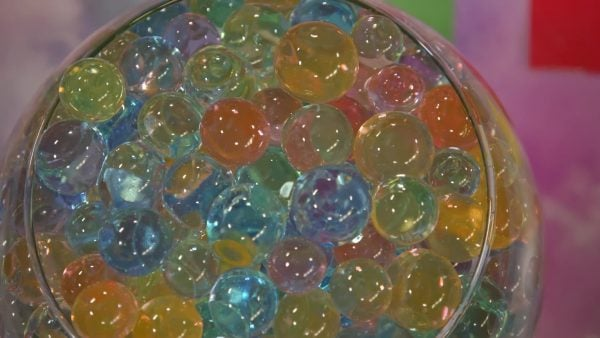If each packet contains 132 jelly balls and we have 3 packets, how many jelly balls are there together? First, we line up the numbers by place value, with the smaller number on the bottom. So, we write 3 under the 2 in 132. Then we draw a line under the bottom number and a multiplication symbol on the left. We start by multiplying 3 by the digit in the ones place: 3 × 2 is 6. We write the 6 in the ones place under the line. Next, we multiply the 3 by the digit in the tens place: 3 × 3 = 9, so we write a 9 in the tens place under the line. Remember that this means 3 × 3 tens, so 9 means 9 tens. Finally, we multiply 3 by the digit in the hundreds place: 3 × 1 = 3, so we write a 3 in the hundreds place under the line. The product is 396 jelly balls! Now you try: Use the standard algorithm to multiply: 413 × 2 = ?

Jelly Balls If each packet contains 132 jelly balls and we have 3 packets, how many jelly balls are there together? First, we line up the numbers by place value, with the smaller number on the bottom. So, we write 3 under the 2 in 132. Then we draw a line under the bottom number and a multiplication symbol on the left. We start by multiplying 3 by the digit in the ones place: 3 × 2 is 6. We write the 6 in the ones place under the line. Next, we multiply the 3 by the digit in the tens place: 3 × 3 = 9, so we write a 9 in the tens place under the line. Remember that this means 3 × 3 tens, so 9 means 9 tens. Finally, we multiply 3 by the digit in the hundreds place: 3 × 1 = 3, so we write a 3 in the hundreds place under the line. The product is 396 jelly balls! Now you try: Use the standard algorithm to multiply: 413 × 2 = ?

### Fan mail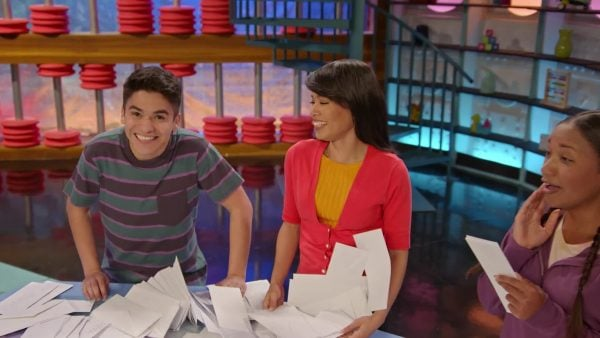Adesina shows April and Marcos how to use the standard algorithm to calculate how much mail is delivered in 3 days, if each day 2,865 pieces of mail are delivered. First, we line up the numbers by place value with 3 on the bottom, and then we multiply each digit, starting with the ones place and working our way to the left. 3 × 5 = 15. We write 5 below the line in the ones place and we place the 1 above the 6 in the tens place to represent the 1 ten in 15. That means that after we multiply by the digit in the tens place, we need to add a 1 ten to the total number of tens. 3 × 6 tens + 1 ten is 19 tens, so we write 9 below the line in the tens place, and we regroup 10 tens as 1 hundred by writing it above the 8 in the hundreds place. Now when we multiply 3 by 8, meaning 3 × 8 hundreds, we add 1, meaning 1 hundred, to the answer. 3 × 8 hundreds + 1 hundred is 25 hundreds, so we write 5 in the hundreds place below the line, and we regroup 20 hundreds as 2 thousands by writing it above the thousands place. Finally, we multiply 3 by 2 thousands and we add the regrouped 2 thousands to get 8 thousands. We can write 8 under the line in the thousands place. The final answer is 8,595. Now you try: 3,719 × 4 = ?

Fan mail Adesina shows April and Marcos how to use the standard algorithm to calculate how much mail is delivered in 3 days, if each day 2,865 pieces of mail are delivered. First, we line up the numbers by place value with 3 on the bottom, and then we multiply each digit, starting with the ones place and working our way to the left. 3 × 5 = 15. We write 5 below the line in the ones place and we place the 1 above the 6 in the tens place to represent the 1 ten in 15. That means that after we multiply by the digit in the tens place, we need to add a 1 ten to the total number of tens. 3 × 6 tens + 1 ten is 19 tens, so we write 9 below the line in the tens place, and we regroup 10 tens as 1 hundred by writing it above the 8 in the hundreds place. Now when we multiply 3 by 8, meaning 3 × 8 hundreds, we add 1, meaning 1 hundred, to the answer. 3 × 8 hundreds + 1 hundred is 25 hundreds, so we write 5 in the hundreds place below the line, and we regroup 20 hundreds as 2 thousands by writing it above the thousands place. Finally, we multiply 3 by 2 thousands and we add the regrouped 2 thousands to get 8 thousands. We can write 8 under the line in the thousands place. The final answer is 8,595. Now you try: 3,719 × 4 = ?

### Eggs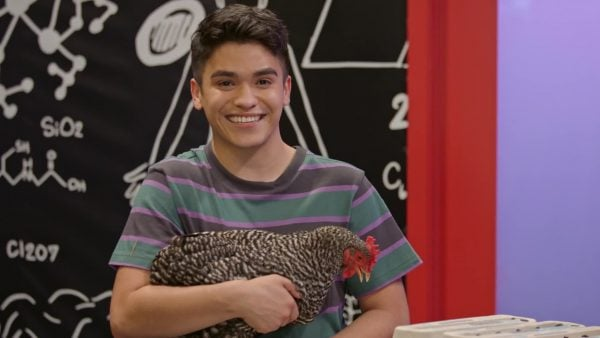Adesina, April, and Marcos use the algorithm to find out how many eggs there are in 24 cartons that contain 12 eggs each. To start, we line up the numbers by place value, with the bigger number on top. First, we need to multiply the ones place of the bottom number by each digit in the top number, and then we must do the same thing with the tens place. First, multiply 2 by 4, which is 8. We write 8 underneath the line in the ones place. Next, we multiply 2 by the 2 in the tens place. 2 × 2 = 4, so we write 4 in the tens place under the line. Our first partial product is 48. Now we multiply 24 by the tens place, 1. Since we are multiplying by tens, we place a 0 in the ones place. Multiply 1 ten by 4 to get 4 tens. We write 4 in the tens place. Then we multiply 1 ten by 2 tens to get 2 hundreds, so we place 2 in the hundreds place. Our second partial product is 240. Notice that all the same place values are lined up in our partial products. Add the partial products together to get the total product. 48 + 240 = 288. There are 288 eggs. Now you try: 31 × 23 = ?

Eggs Adesina, April, and Marcos use the algorithm to find out how many eggs there are in 24 cartons that contain 12 eggs each. To start, we line up the numbers by place value, with the bigger number on top. First, we need to multiply the ones place of the bottom number by each digit in the top number, and then we must do the same thing with the tens place. First, multiply 2 by 4, which is 8. We write 8 underneath the line in the ones place. Next, we multiply 2 by the 2 in the tens place. 2 × 2 = 4, so we write 4 in the tens place under the line. Our first partial product is 48. Now we multiply 24 by the tens place, 1. Since we are multiplying by tens, we place a 0 in the ones place. Multiply 1 ten by 4 to get 4 tens. We write 4 in the tens place. Then we multiply 1 ten by 2 tens to get 2 hundreds, so we place 2 in the hundreds place. Our second partial product is 240. Notice that all the same place values are lined up in our partial products. Add the partial products together to get the total product. 48 + 240 = 288. There are 288 eggs. Now you try: 31 × 23 = ?

### Collecting coins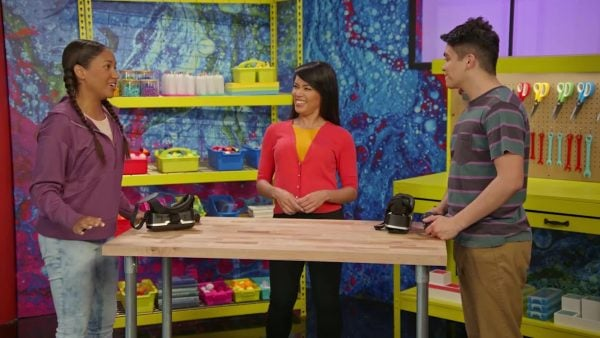April and Marcos collected 145 coins in each of 37 levels of a video game. They want to use the algorithm to calculate how many coins they have altogether. As usual, 145 is placed above 37, aligned by place value. Starting with the ones place, we multiply 7 × 5 = 35. Write the 5 in the ones place, and regroup the 3 above the tens place. Next, we multiply 7 × 4 = 28, plus the 3 we regrouped, to make 31. We write 1 in the tens place and regroup 3 above the hundreds place. Then we find 7 × 1 = 7, and we add the 3 we regrouped to get 10. The 0 goes in the hundreds place and the 1 goes in the thousands place. Our first partial product is 1,015. Now, we need to multiply 145 by the digit in the tens place. Since we have no ones in our second product, we write a 0 in the ones place below our first partial product. Then, start by multiplying 3 × 5 = 15. Place the 5 in the tens place and the 1 is regrouped above the hundreds place. Next, multiply 3 × 4 = 12. Add the 1 that was regrouped to get a total of 13. The 3 goes in the hundreds place, and the 1 is regrouped to the thousands place. Finally, we find 3 × 1 = 3. Add the 1 that we regrouped for a total of 4. The 4 goes in the thousands place. The second partial product is 4,350. Add the partial products together to find the total product: 1,015 + 4,350 = 5,365 coins! Now you try: 1,589 × 43 = ?

Collecting coins April and Marcos collected 145 coins in each of 37 levels of a video game. They want to use the algorithm to calculate how many coins they have altogether. As usual, 145 is placed above 37, aligned by place value. Starting with the ones place, we multiply 7 × 5 = 35. Write the 5 in the ones place, and regroup the 3 above the tens place. Next, we multiply 7 × 4 = 28, plus the 3 we regrouped, to make 31. We write 1 in the tens place and regroup 3 above the hundreds place. Then we find 7 × 1 = 7, and we add the 3 we regrouped to get 10. The 0 goes in the hundreds place and the 1 goes in the thousands place. Our first partial product is 1,015. Now, we need to multiply 145 by the digit in the tens place. Since we have no ones in our second product, we write a 0 in the ones place below our first partial product. Then, start by multiplying 3 × 5 = 15. Place the 5 in the tens place and the 1 is regrouped above the hundreds place. Next, multiply 3 × 4 = 12. Add the 1 that was regrouped to get a total of 13. The 3 goes in the hundreds place, and the 1 is regrouped to the thousands place. Finally, we find 3 × 1 = 3. Add the 1 that we regrouped for a total of 4. The 4 goes in the thousands place. The second partial product is 4,350. Add the partial products together to find the total product: 1,015 + 4,350 = 5,365 coins! Now you try: 1,589 × 43 = ?

## MULTIPLICATION USING THE STANDARD ALGORITHM VOCABULARY

Product
The final answer in a multiplication problem.
Partial product
Part of the product that is added to another part to form the product.
Area model
A multiplication method that uses rectangles and breaks numbers up by place value.
Algorithm
A set of rules and steps that you follow to perform a calculation.
Place value
The value of each digit in a number.
Digit
Any numeral between 0 and 9 that forms part of a number.

## MULTIPLICATION USING THE STANDARD ALGORITHM DISCUSSION QUESTIONS

### Which is better, the area model or the multiplication algorithm?

Both help us in different ways. The area model helps us to understand what we are multiplying and to visualize the result. The algorithm is faster and can help us multiply large numbers more quickly, but we should only use it if we understand what it represents.

### What does partial product mean? How do we use partial products?

Sometimes when we use the multiplication algorithm, we end up with two or more partial products. These are part of the answer, and need to be added together to get the final product.

### Giovanni uses the algorithm to multiply 352 by 7. First he finds 7 × 2. When he finds 7 × 5, what is he really multiplying? When he multiplies 7 × 3, what is he really multiplying?

Multiplying 7 × 2 is 7 × 2 ones, multiplying 7 × 5 is 7 × 5 tens, multiplying 7 × 3 is 7 × 3 hundreds.

### Fiona uses the algorithm to multiply 17 × 8. First she multiplies 8 × 7 and gets 56. Where should she put the 5, and what happens to the 6?

The 5 goes under the ones place and the 6 is written above the tens place, to be added to the product after multiplying by 8 × 1.

### Victor says he is finished multiplying 253 × 17. What did he forget to do?

He forgot to write a 0 on the ones place of the second partial product. We need to put 0 in the ones place because there are 0 ones in the partial product. He also forgot to add the partial products together to get the final product.
X

## Success

We’ve sent you an email with instructions how to reset your password.
Ok

## Choose Your Free Trial Period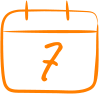### 7 DaysContinue to Lessons### 90 DaysGet 90 days free by inviting other teachers to try it too.

Share with Teachers

## Get 90 Days Free

### By inviting 4 other teachers to try it too.

4 required

Skip, I will use a 7 days free trial

## Thank You!

Enjoy your free 90 days trial